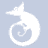quizzes  Forums

# Regression1

5 played - 11 yrs ago
Regression1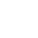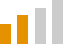11 QUESTIONS
58%1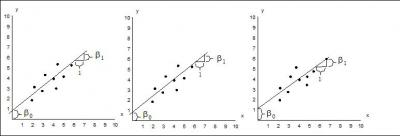An experiment is performed 3 times. Each time a model is fit. This means we generate how many slopes? How many y-intercepts?

2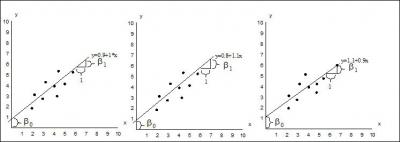Using the question above, is it accurate to say that the slope and the y-intercept are both random variables? That is, they vary as we repeat our experiment and fit the model again and again?

3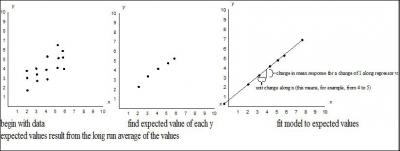When we fit a model, the line gives what exactly?

4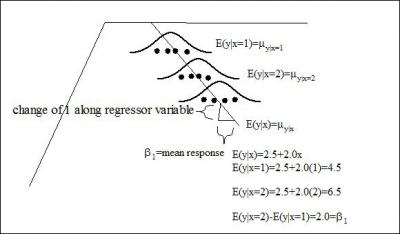The picture below shows what happens when we fit a model. Is this true or false?

5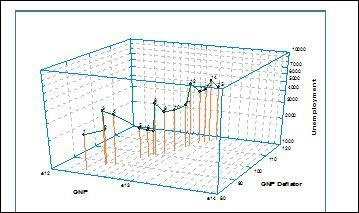It looks as though the two variables are col-linear. Which means what exactly?

6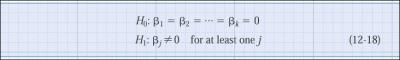The hypothesis stated below are correct for a modeling using a multiple regressors.

7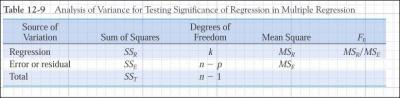The table shown below is correct. That is, it shows the correct entries for multiple regression. n-p=n-(k+1)=n-k-1 k is number of predictors

8
Prediction intervals are wider then confidence intervals because they depend on the current estimate of the mean and the spread of the data. That is, we fit a model and reach in a grab a new datapoint

9
If I conduct a regression analysis, and compute t stats. for each regression coefficient, then the square of these is what?

10
It's possible to conduct partial regression tests on the contribution made by several predictors, given that several others are already in the model.

11
I fit a model first with 1 regressor and then 2. R^2 adjusted increases. This means what?